# GMAT Math : Sets

## Example Questions

← Previous 1 3

### Example Question #1 : Arithmetic

Which set is NOT equal to the other sets?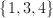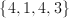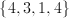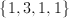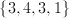Explanation:

Order and repetition do NOT change a set.  Therefore, the set we want to describe contains the numbers 1, 3, and 4.  The only set that doesn't contain all 3 of these numbers is, so it is the set that does not equal the rest of the sets.

### Example Question #1 : Understanding Arithmetic Sets

Given the sets A = {2, 3, 4, 5} and B = {3, 5, 7}, what is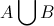?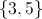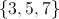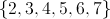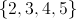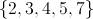Explanation:

We are looking for the union of the sets.  That means we want the elements of A OR B.

So= {2, 3, 4, 5, 7}.

### Example Question #3 : Sets

Given the set= {2, 3, 4, 5}, what is the value of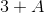?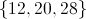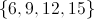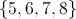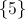Explanation:

We need to add 3 to every element in.

Then: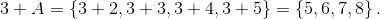### Example Question #1 : Understanding Arithmetic Sets

There exists two setsand.= {1, 4} and= {3, 4, 6}.  What is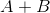?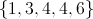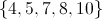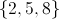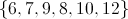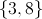Explanation:

Add each element ofto each element of.= {1 + 3, 1 + 4, 1 + 6, 4 + 3, 4 + 4, 4 + 6} = {4, 5, 7, 8, 10}

### Example Question #1 : Sets

How many functions map from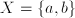to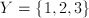?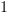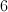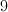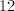Explanation:

There are three choices for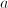(1, 2, and 3), and similarly there are three choices for(also 1, 2, and 3).  Together there are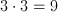possible functions from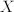to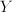.  Remember to multiply, NOT add.

### Example Question #1 : Arithmetic

How many elements are in a set from which exactly 768 unique subsets can be formed?It is not possible to form exactly 768 unique subsets.It is not possible to form exactly 768 unique subsets.

Explanation:

The number of subsets that can be formed from a set withelements is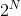. However,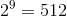and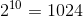, so there is no integerfor which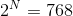. Therefore, a set with exactly 768 elements cannot exist.

### Example Question #1 : Sets

Let the univeraal set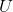be the set of all positive integers.

Define the sets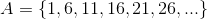,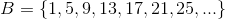,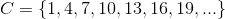.

If the elements in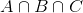were ordered in ascending order, what would be the fourth element?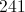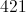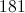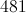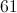Explanation: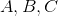are the sets of all positive integers that are one greater than a multiple of five, four, and three, respectively. Therefore, for a number to be in all three sets, and subsequently,, the number has to be one greater than a number that is a multiple of five, four, and three. Since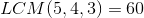, the number has to be one greater than a multiple of 60. The first four numbers that fit this description are 1, 61, 121, and 181, the last of which is the correct choice.

### Example Question #1 : Arithmetic

A six-sided die is rolled, and a coin is flipped. If the coin comes up heads, the roll is considered to be the number that appears face up on the die; if the coin comes up tails, the outcome is considered to be twice that number. What is the sample space of the experiment?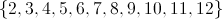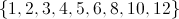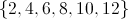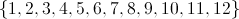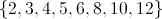Explanation:

If heads comes up on the coin, the number on the die is recorded. This can be any element of the set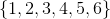.

If tails comes up on the coin, twice the number on the die is recorded. This can be twice any element of the set- that is, any element of the set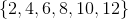.

The sample space is the union of these two sets: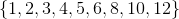### Example Question #1 : Arithmetic

A six-sided die is rolled, and a coin is flipped. If the coin comes up heads, the roll is considered to be the number that appears face up on the die; if the coin comes up tails, the outcome is considered to be half that number, with any fraction simply thrown out. What is the sample space of the experiment?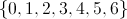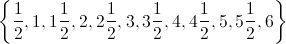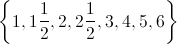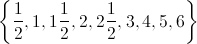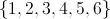Explanation:

If heads comes up on the coin, the number on the die is recorded. This can be any element of the set.

If tails comes up on the coin, half the number on the die is recorded, and any fraction is thrown out. Half the elements of the setcomprise the set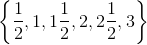, but since we are throwing out the fractional parts, this becomes the set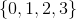The sample space is the union of these two sets, which is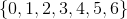.

### Example Question #1 : Arithmetic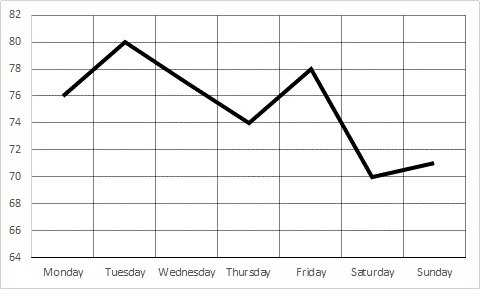Refer to the above graph, which shows the peak temperature in Smithville for each of seven days in a one-week period.

Between which two consecutuve days did the peak temperature see its greatest decrease?

Thursday to Friday

Tuesday to Wednesday

Wednesday to Thursday

Friday to Saturday

Monday to Tuesday